Open in App
Not now

# Detecting Spam Emails Using Tensorflow in Python

• Difficulty Level : Expert
• Last Updated : 14 Jan, 2023

Spam messages refer to unsolicited or unwanted messages/emails that are sent in bulk to users. In most messaging/emailing services, messages are detected as spam automatically so that these messages do not unnecessarily flood the users’ inboxes. These messages are usually promotional and peculiar in nature. Thus, it is possible for us to build ML/DL models that can detect Spam messages.

## Detecting Spam Emails Using Tensorflow in Python

In this article, we’ll build a TensorFlow-based Spam detector; in simpler terms, we will have to classify the texts as Spam or Ham. This implies that Spam detection is a case of a Text Classification problem. So, we’ll be performing EDA on our dataset and building a text classification model.

## Importing Libraries and Dataset

Python libraries make it very easy for us to handle the data and perform typical and complex tasks with a single line of code.

• Pandas – This library helps to load the data frame in a 2D array format and has multiple functions to perform analysis tasks in one go.
• Numpy – Numpy arrays are very fast and can perform large computations in a very short time.
• Matplotlib/Seaborn/Wordcloud This library is used to draw visualizations.
• NLTK – Natural Language Tool Kit provides various functions to process the raw textual data.

## Python3

 `#Importing necessary libraries for EDA``import` `numpy as np``import` `pandas as pd``import` `matplotlib.pyplot as plt``import` `seaborn as sns` `import` `string``import` `nltk``from` `nltk.corpus ``import` `stopwords``from` `wordcloud ``import` `WordCloud``nltk.download(``'stopwords'``)` `#Importing libraries necessary for Model Building and Training``import` `tensorflow as tf``from` `tensorflow.keras.preprocessing.text ``import` `Tokenizer``from` `tensorflow.keras.preprocessing.sequence ``import` `pad_sequences``from` `sklearn.model_selection ``import` `train_test_split`  `import` `warnings``warnings.filterwarnings(``'ignore'``)`

Now let’s load the dataset into a pandas data frame and look at the first five rows of the dataset.

## Python3

 `df ``=` `pd.read_csv(``'emails.csv'``)``df.head()`

Output: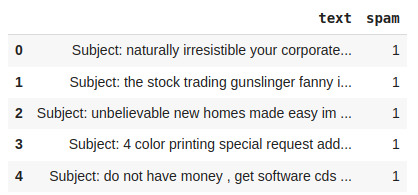First five rows of the dataset

To check how many such tweets data we have let’s print the shape of the data frame.

## Python3

 `df.shape`

Output:

`(5728, 2)`

For a better understanding, we’ll plot these counts:

## Python3

 `sns.countplot(data[``'spam'``])``plt.show()`

Output: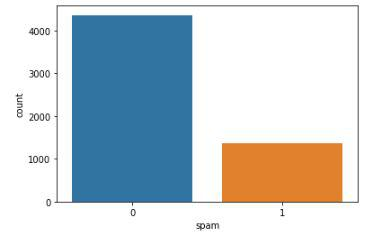Count plot for the spam labels

We can clearly see that number of samples of Ham is much more than that of Spam which implies that the dataset we are using is imbalanced.

## Python3

 `# Downsampling to balance the dataset``ham_msg ``=` `data[data.spam ``=``=` `0``]``spam_msg ``=` `data[data.spam ``=``=` `1``]``ham_msg ``=` `ham_msg.sample(n ``=` `len``(spam_msg), random_state``=``42``)` `# Plotting the counts of down sampled dataset``balanced_data ``=` `ham_msg.append(spam_msg).reset_index(drop ``=` `True``)``plt.figure(figsize ``=` `(``8``, ``6``))``sns.countplot(balanced_data.spam)``plt.title(``'Distribution of Ham and Spam email messages after downsampling'``)``plt.xlabel(``'Message types'``)`

Output: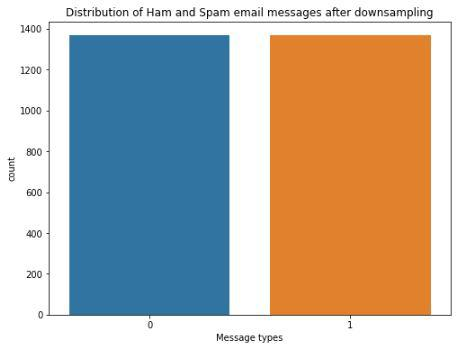## Text Preprocessing

Textual data is highly unstructured and need attention on many aspects like:

Although removing data means loss of information but we need to do this to make the data perfect to feed into a machine learning model.

## Python3

 `data[``'text'``] ``=` `data[``'text'``].``str``.replace(``'Subject'``, '')``data.head()`

Output: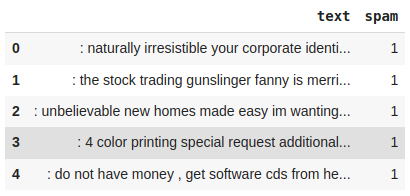## Python3

 `punctuations_list ``=` `string.punctuation``def` `remove_punctuations(text):``    ``temp ``=` `str``.maketrans('``', '``', punctuations_list)``    ``return` `text.translate(temp)` `df[``'text'``]``=` `df[``'text'``].``apply``(``lambda` `x: remove_punctuations(x))``df.head()`

Output: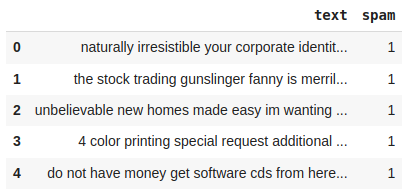Dataset after removal of punctuation’s

The below function is a helper function that will help us to remove the stop words.

## Python3

 `def` `remove_stopwords(text):``    ``stop_words ``=` `stopwords.words(``'english'``)` `    ``imp_words ``=` `[]` `    ``# Storing the important words``    ``for` `word ``in` `str``(text).split():``        ``word ``=` `word.lower()` `        ``if` `word ``not` `in` `stop_words:``            ``imp_words.append(word)` `    ``output ``=` `" "``.join(imp_words)` `    ``return` `output`  `df[``'text'``] ``=` `df[``'text'``].``apply``(``lambda` `text: remove_stopwords(text))``df.head()`

Output: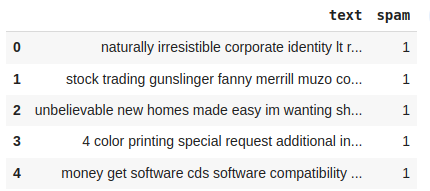Dataset after removal of stop words

Word cloud is a text visualisation tool that help’s us to get insights into the most frequent words present in the corpus of the data.

## Python3

 `def` `plot_word_cloud(data, typ):``    ``email_corpus ``=` `" "``.join(data[``'text'``])` `    ``plt.figure(figsize``=``(``10``, ``10``))``    ``wc ``=` `WordCloud(background_color``=``'white'``,``                   ``max_words``=``100``,``                   ``width``=``200``,``                   ``height``=``100``,``                   ``collocations``=``False``).generate(email_corpus)` `    ``plt.title(f``'WordCloud for {typ} emails.'``, fontsize``=``15``)``    ``plt.axis(``'off'``)``    ``plt.imshow(wc)``    ``plt.show()``    ``print``()`  `plot_word_cloud(df[df[``'spam'``] ``=``=` `0``], typ``=``'Non - Spam'``)``plot_word_cloud(df[df[``'spam'``] ``=``=` `1``], typ``=``'Spam'``)`

Output:Word cloud for the two class of data

## Word2Vec Conversion

We cannot feed words to a machine learning model because they work on numbers only. So, first, we will convert the our words to vectors with the token id’s to the corresponding words and after padding them our textual data will arrive to a stage where we can feed it to a model.

## Python3

 `#train test split``train_X, test_X, train_Y, test_Y ``=` `train_test_split(balanced_data[``'text'``],``                                                    ``balanced_data[``'spam'``],``                                                    ``test_size ``=` `0.2``,``                                                    ``random_state ``=` `42``)`

We have fitted the tokenizer on our training data we will use it to convert the training and validation data both to vectors.

## Python3

 `# training the tokenizer``token``=``Tokenizer()``token.fit_on_texts(train_X)` `#Generating token embeddings``Training_seq ``=` `token.texts_to_sequences(train_X)``Training_pad ``=` `pad_sequences(Training_seq,``                             ``maxlen ``=` `50``,``                             ``padding ``=` `'post'``,``                             ``truncating ``=` `'post'``)` `Testing_seq ``=` `token.texts_to_sequences(test_X)``Testing_pad ``=` `pad_sequences(Testing_seq,``                            ``maxlen ``=` `50``,``                            ``padding ``=` `'post'``,``                            ``truncating ``=` `'post'``)`

## Model Development and Evaluation

We will implement a Sequential model which will contain the following parts:

• Three Embedding Layers to learn a featured vector representations of the input vectors.
• A LSTM layer to identify useful patterns in the sequence.
• Then we will have one fully connected layer.
• The final layer is the output layer which outputs probabilities for the two classes.

## Python3

 `# Building the Model``model ``=` `tf.keras.models.Sequential()``model.add(tf.keras.layers.Embedding(max_words, ``32``,``                                    ``input_length``=``50``))``model.add(tf.keras.layers.LSTM(``4``))``model.add(tf.keras.layers.Dense(``32``, activation``=``'relu'``))``model.add(tf.keras.layers.Dense(``1``, activation``=``'sigmoid'``))`

While compiling a model we provide these three essential parameters:

• optimizer – This is the method that helps to optimize the cost function by using gradient descent.
• loss – The loss function by which we monitor whether the model is improving with training or not.
• metrics – This helps to evaluate the model by predicting the training and the validation data.

## Python3

 `model.``compile``(loss ``=` `tf.keras.losses.BinaryCrossentropy(from_logits ``=` `True``),``              ``metrics ``=` `[``'accuracy'``],``              ``optimizer ``=` `'adam'``)`

### Callback

Callbacks are used to check whether the model is improving with each epoch or not. If not then what are the necessary steps to be taken like ReduceLROnPlateau decreases learning rate further. Even then if model performance is not improving then training will be stopped by EarlyStopping. We can also define some custom callbacks to stop training in between if the desired results have been obtained early.

## Python3

 `from` `keras.callbacks ``import` `EarlyStopping, ReduceLROnPlateau` `es ``=` `EarlyStopping(patience``=``3``,``                   ``monitor ``=` `'val_accuracy'``,``                   ``restore_best_weights ``=` `True``)` `lr ``=` `ReduceLROnPlateau(patience ``=` `2``,``                       ``monitor ``=` `'val_loss'``,``                       ``factor ``=` `0.5``,``                       ``verbose ``=` `0``)`

Let us now train the model:

## Python3

 `history ``=` `model.fit(Training_pad, train_Y,``                    ``validation_data ``=` `(Testing_pad, test_Y),``                    ``epochs ``=` `30``,``                    ``verbose ``=` `1``,``                    ``batch_size ``=` `32``,``                    ``callbacks ``=` `[lr, es])`

Output:

```Epoch 1/30
69/69 [==============================] - 5s 34ms/step - loss: 0.6552 - accuracy: 0.7491 - val_loss: 0.5573 - val_accuracy: 0.8394 - lr: 0.0010
Epoch 2/30
69/69 [==============================] - 2s 24ms/step - loss: 0.3301 - accuracy: 0.9333 - val_loss: 0.1511 - val_accuracy: 0.9672 - lr: 0.0010
Epoch 3/30
69/69 [==============================] - 2s 25ms/step - loss: 0.0777 - accuracy: 0.9872 - val_loss: 0.1403 - val_accuracy: 0.9544 - lr: 0.0010
Epoch 4/30
69/69 [==============================] - 2s 25ms/step - loss: 0.0437 - accuracy: 0.9909 - val_loss: 0.1087 - val_accuracy: 0.9726 - lr: 0.0010
Epoch 5/30
69/69 [==============================] - 2s 25ms/step - loss: 0.0278 - accuracy: 0.9954 - val_loss: 0.1028 - val_accuracy: 0.9708 - lr: 0.0010
Epoch 6/30
69/69 [==============================] - 2s 26ms/step - loss: 0.0210 - accuracy: 0.9968 - val_loss: 0.1050 - val_accuracy: 0.9745 - lr: 0.0010
Epoch 7/30
69/69 [==============================] - 2s 26ms/step - loss: 0.0147 - accuracy: 0.9982 - val_loss: 0.1160 - val_accuracy: 0.9745 - lr: 0.0010
Epoch 8/30
69/69 [==============================] - 2s 25ms/step - loss: 0.0137 - accuracy: 0.9982 - val_loss: 0.1202 - val_accuracy: 0.9726 - lr: 5.0000e-04
Epoch 9/30
69/69 [==============================] - 2s 25ms/step - loss: 0.0133 - accuracy: 0.9982 - val_loss: 0.1263 - val_accuracy: 0.9726 - lr: 5.0000e-04```

Now, let’s evaluate the model on the validation data.

## Python3

 `model.evaluate(Testing_pad, test_Y)`

Output:

```18/18 [==============================] - 0s 6ms/step - loss: 0.1050 - accuracy: 0.9745
[0.10502833873033524, 0.974452555179596]```

Thus, the training accuracy turns out to be 97.44% which is quite satisfactory.

### Model Evaluation Results

Having trained our model, we can plot a graph depicting the variance of training and validation accuracies with the no. of epochs.

## Python3

 `plt.plot(history.history[``'accuracy'``])``plt.plot(history.history[``'val_accuracy'``])``plt.title(``'model accuracy'``)``plt.ylabel(``'accuracy'``)``plt.xlabel(``'epoch'``)`

Output: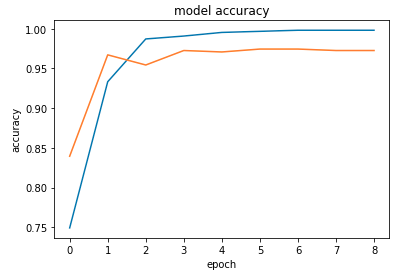Model’s accuracy epoch by epoch

My Personal Notes arrow_drop_up